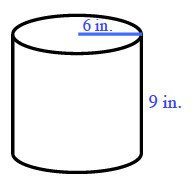### Home > GC > Chapter 9 > Lesson 9.1.4 > Problem9-40

9-40.

Draw a cylinder on your paper. Assume the radius of the cylinder is $6$ inches and the height is $9$ inches.

1. What is the surface area of the cylinder? What is the volume?

The surface area of a cylinder is the sum of the areas of its top base, its bottom base, and its lateral surface.

$\text{Surface area of top base}=36\pi\text{ in.}^2$

$\text{Surface area of bottom base}=\text{Surface area of top base}$

$\text{Area of lateral surface}=(12\pi)(9)=108\pi\text{ in.}$$\text{SA} = 2 (36π) + 108π = 180π\text{ in.}²$

$\text{V} = 9(36π) = 324π ≈ 1,017.9\text{ in.}³$

2. If the cylinder is enlarged with a linear scale factor of $3$, what is the volume of the enlarged cylinder? How do you know?

How does increasing the scale by $3$ affect the volume? What must you multiply the volume by to determine the new volume?